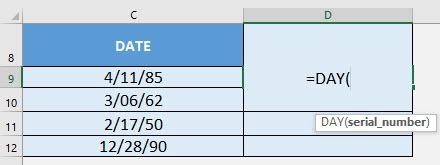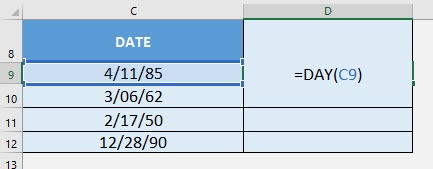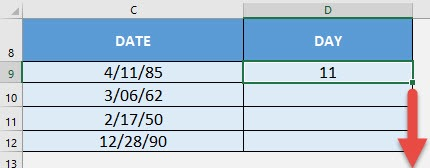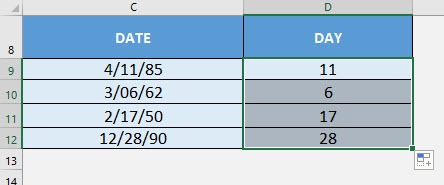What does it do?

Gets the day from the date

Formula breakdown:

=DAY(serial_number)

What it means:

=DAY(date wherein the day will be extracted from)

I recall wherein I had a lot of dates and I wanted to extract the day of the dates one-by-one… It was too much of a hassle! Thankfully there is Excel’s DAY Formula to do that for me!

I explain how you can do this below:STEP 1: We need to enter the DAY function in a blank cell:

## =DAY(STEP 2: The DAY arguments:

## serial_number

What is the date that you want to extract the day from?

Select the cell containing the date:

## =DAY(C9)Apply the same formula to the rest of the cells by dragging the lower right corner downwards.You now have your days extracted!How to Use the DAY Formula in Excel